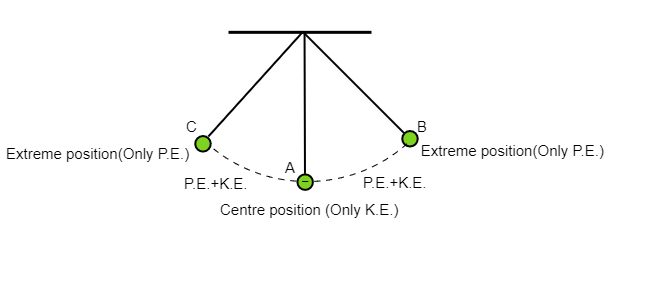# with pendulum as an example explain the law of conservation of energy.

Law of conservation of energy with the help of a pendulum:Law of conservation of energy states that energy neither can be created nor destroyed, it can be only transformed from one form to another form of energy.

A simple pendulum is an example of conservation of energy. It can be understood as:

1. At center position B only Potential energy it has.

2. While moving down towards center A Potential energy starts decreasing and kinetic energy starts increasing.

3. At center A Potential energy is zero and the bob of pendulum has only kinetic energy.

4. While the bob moving from center A to Extreme position B kinetic energy starts decreasing and potential energy starts increasing.

5. At extreme position C the pendulum has only potential energy.

Updated on: 10-Oct-2022

315 Views